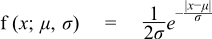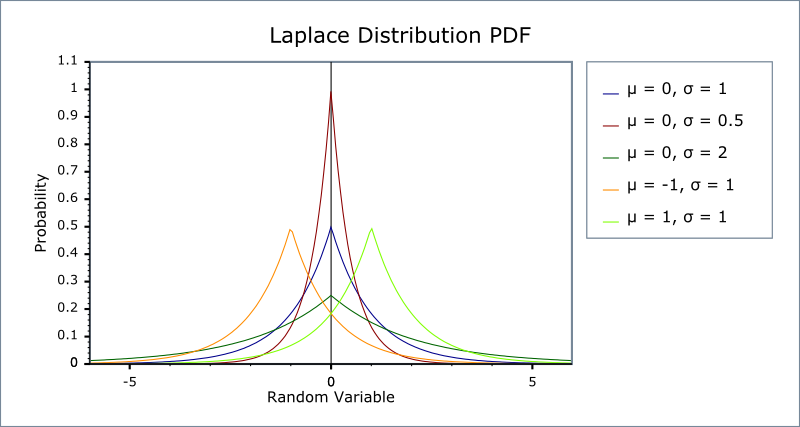#Boost C++ Libraries

...one of the most highly regarded and expertly designed C++ library projects in the world.

This is the documentation for an old version of boost. Click here for the latest Boost documentation.
##### Laplace Distribution

`#include <boost/math/distributions/laplace.hpp>`

```namespace boost{ namespace math{

template <class RealType = double,
class Policy   = policies::policy<> >
class laplace_distribution;

typedef laplace_distribution<> laplace;

template <class RealType, class Policy>
class laplace_distribution
{
public:
typedef RealType value_type;
typedef Policy   policy_type;
// Construct:
laplace_distribution(RealType location = 0, RealType scale = 1);
// Accessors:
RealType location()const;
RealType scale()const;
};

}} // namespaces
```

Laplace distribution is the distribution of differences between two independent variates with identical exponential distributions (Abramowitz and Stegun 1972, p. 930). It is also called the double exponential distribution.

For location parameter μ   and scale parameter σ   it is defined by the probability density function:The location and scale parameters are equivalent to the mean and standard deviation of the normal or Gaussian distribution.

The following graph illustrates the effect of the parameters μ   and σ   on the PDF. Note that the range of the random variable remains [-∞,+∞] irrespective of the value of the location parameter:##### Member Functions
```laplace_distribution(RealType location = 0, RealType scale = 1);
```

Constructs a laplace distribution with location location and scale scale.

The location parameter is the same as the mean of the random variate.

The scale parameter is proportional to the standard deviation of the random variate.

Requires that the scale parameter is greater than zero, otherwise calls domain_error.

```RealType location()const;
```

Returns the location parameter of this distribution.

```RealType scale()const;
```

Returns the scale parameter of this distribution.

##### Non-member Accessors

All the usual non-member accessor functions that are generic to all distributions are supported: Cumulative Distribution Function, Probability Density Function, Quantile, Hazard Function, Cumulative Hazard Function, mean, median, mode, variance, standard deviation, skewness, kurtosis, kurtosis_excess, range and support.

The domain of the random variable is [-∞,+∞].

##### Accuracy

The laplace distribution is implemented in terms of the standard library log and exp functions and as such should have very small errors.

##### Implementation

In the following table μ is the location parameter of the distribution, σ is its scale parameter, x is the random variate, p is the probability and its complement q = 1-p.

Function

Implementation Notes

pdf

Using the relation: pdf = e-abs(x-μ) / σ / (2 * σ)

cdf

Using the relations:

x < μ : p = e(x-μ)/σ / σ

x >= μ : p = 1 - e(μ-x)/σ / σ

cdf complement

Using the relation:

-x < μ : q = e(-x-μ)/σ / σ

-x >= μ : q = 1 - e(μ+x)/σ / σ

quantile

Using the relations:

p < 0.5 : x = μ + σ * log(2*p)

p >= 0.5 : x = μ - σ * log(2-2*p)

quantile from the complement

Using the relation:

q > 0.5: x = μ + σ*log(2-2*q)

q <=0.5: x = μ - σ*log( 2*q )

mean

μ

variance

2 * σ2

mode

μ

skewness

0

kurtosis

6

kurtosis excess

3